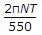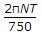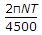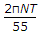# Civil Engineering - Strength of Materials

31.
If a shaft is rotating N revolutions per minute with an applied torque T kg-m, the horse power being transmitted by the shaft, isnone of these.
Explanation:
No answer description is available. Let's discuss.

32.
If the width of a simply supported beam carrying an isolated load at its centre is doubled, the deflection of the beam at the centre is changed by
1/2
1/8
2
8
4
Explanation:
No answer description is available. Let's discuss.

33.
For a simply supported beam of length L, the bending moment M is described as M = a (x - x3/L2), 0 ≤ x < L; where a is a constant. The shear force will be zero at
the supports
x = L/2
x = L/3
x = L/3
Explanation:
No answer description is available. Let's discuss.

34.
The deflection of any rectangular beam simply supported, is
directly proportional to its weight
inversely proportional to its width
inversely proportional to the cube of its depth
directly proportional to the cube of its length
none of these.